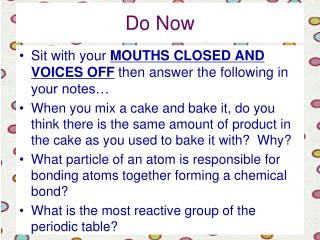DownloadDownload PresentationDo Now

# Do Now

Download Presentation## Do Now

- - - - - - - - - - - - - - - - - - - - - - - - - - - E N D - - - - - - - - - - - - - - - - - - - - - - - - - - -
##### Presentation Transcript

1. Do Now • Sit with your MOUTHS CLOSED AND VOICES OFF then answer the following in your notes… • When you mix a cake and bake it, do you think there is the same amount of product in the cake as you used to bake it with? Why? • What particle of an atom is responsible for bonding atoms together forming a chemical bond? • What is the most reactive group of the periodic table?

2. Chemical Equations Putting chemical changes into words http://app.discoveryeducation.com/techbook2:concept/view/guidConceptId/22cc81ed-8bd3-4b53-8964-18551be0242d/guidUnitId/1ef90b7b-c4ee-40d5-bcdc-ff4f708f8873#/tab=model-lesson-tab&page=1&subTab=sessions1and2 Traits of chemical reactions

3. A chemical equation is a symbolic representation of a chemical reaction.

4. Equation Example: • The burning of methane gas in oxygen is: • CH4 + 2 O2 → CO2 + 2 H2O

5. Review:Element Symbols • All elements are represented by a 1 or 2 letter symbol • For example • C = Carbon • Ne = Neon • O = Oxygen • The symbols are shown on the periodic table

6. Chemical Formulas • Shows the elements & number of atoms of each element in a molecule • H2SO4 • Elements • Hydrogen; 2 atoms • Sulfur: 1 atom • Oxygen: 4 atoms • 7 atoms total per each molecule Subscript

7. Coefficients • A formula may begin with a number. • If there is no number, then “1” is understood to be in front of the formula. • This number is called the coefficient. • The coefficient represents the number of molecules of that compound or atom needed in the reaction. • For example: • 2H2SO4 – 2 molecules of Sulfuric Acid • Which means there are how many of each atom?

8. Coefficients • 2H2SO4 – 2 molecules of Sulfuric Acid • A coefficient is distributed to ALL elements in a compound • 2 – H2 (for a total of 4 H atoms) • 2 – S (for a total of 2 S atoms) • 2 – O4 (for a total of 8 O atoms)

9. Reading Chemical Equations • Each side of an equation represents a combination of chemicals. • The combination is written as a set of chemical formulas, separated by + symbols. CH4 + 2 O2 → CO2 + 2 H2O Coefficient

10. Reading Chemical Equations • The two sides of the equation are separated by an arrow. • The combination of chemicals before the reaction are on the left side of the arrow are known as the reactants • The right side indicates the combination of chemicals after the reaction and these are known as the products

11. For Example: • In this reaction, sodium (Na) and oxygen (O2) react to make a single molecule, Na2O Na + O2 → Na2O

12. Balancing Equations • The Law of Conservation of Mass states that in a chemical reaction, the quantity or amount of each element does not change. • This means that each side of the equation must represent the same quantity of each element; in other words have the same number of each kind of atom.

13. To start balancing… • The first step is to count how many atoms you have on each side of the equation. • Fe + O2 → Fe2O3 Left side: Right side: • 1 atom Fe 2 atoms Fe • 2 atoms O 3 atoms O

14. Balancing cont… • The next step is accomplished by introducing the proper numbers or coefficients before each formula. • IMPORTANT NOTE: DO NOT CHANGE THE SUBSCRIPTS!!! • Fe + O2 → Fe2O3 - Show on Board

15. Balancing EquationsNa + O2 → Na2O In order for this equation to be balanced, there must be equal amountof Na on the left hand side and on the right hand side. Right now, there is 1 Na atom on the left but 2 Na atoms on the right. We solve this problem by putting a 2 in front of the Na on the left hand side, Like this: 2Na + O2 → Na2O

16. Balancing Equations2Na + O2 → Na2O There are 2 Na's on the left and 2 Na's on the right. But what about the O? We now must check to see if the O's are balanced on both sides of the equation. On the left hand side there are 2 O's and the right hand side only has one. This is still an unbalanced equation. To fix this we must put a 2 in front of the Na2O on the right hand side. Now our equation reads: 2Na + O2 → 2Na2O

17. Balancing Equations2Na + O2 → 2Na2O Notice that the 2 on the right hand side is "distributed" to both the Na2 and the O. Currently the left hand side of the equation has 2 Na's and 2O's. The right hand side has 4 Na's total and 2 O's. Again, this is a problem, there must be an equal amount of each chemical on both sides. To fix this let's add 2 more Na's on the left side. The equation will now look like this: 4Na + O2 → 2Na2O

18. Your turn… • Balance these equations in your notes • N2 + H2 → NH3 • K + B2O3 → K2O + B • Al(OH)3 + HBr → AlBr3 + H2O • FeS2 + O2 → Fe2O3 + SO2

19. Exit Ticket • In a chemical equation, what does a subscript stand for, an atom or a molecule? • In a chemical equation, what does a coefficient stand for, an atom or a molecule? • What does the law of conservation of mass state?